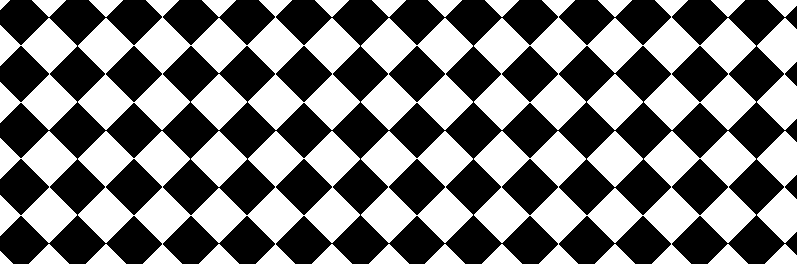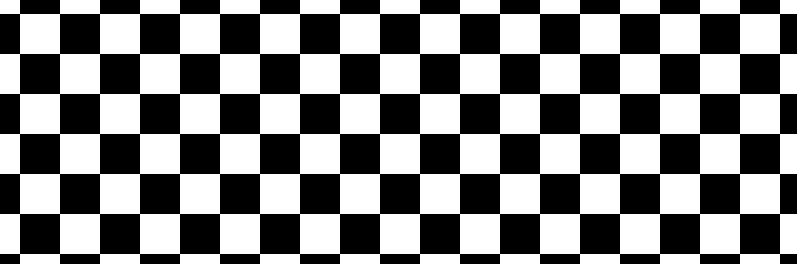Open In App

# How to create Chess pattern background using HTML and CSS ?

Chess patterns are simply certain tactical positions which regularly occur in games. It can be easily design by using the pseudo selector of CSS. This type of pattern can be used to create confusing illusions.

Approach: The approach is to use repeating linear gradient property to create a pattern that will be repeated to the defined height and width.

HTML Code: In this section, we will design the basic structure of the chess pattern.

## HTML

 ```<``html``>`` ` `<``head``>``    ``<``title``>Chess Pattern```` ` `<``body``>``    ``<``div` `class``=``"geeks"``>```` ` ``

CSS Code: For CSS, follow the below given steps.

• Step 1: Set the position of div to fixed and apply some height and width.
• Step 2: Now use before selector and apply repeating linear-gradient property with black and white color and angle set as 45deg.
• Step 3: Now use after selector with the same properties as used in before just change the angle to -45 deg.
• Step 4: Set the mix-blend mode to difference so that it can overlay on the background.

Tip: You can also use 0 deg in before and 90 deg in after to make perfect square chess like boxes. The output of the same will also be attached below.

## CSS

 ``

Complete Code: (With angles 45 deg and -45 deg): It is the combination of the above two sections of code to design a chess pattern.

## HTML

 ```<``html``>`` ` `<``head``>``    ``<``title``>Chess Pattern```` ` `<``style``>``    ``.geeks {``        ``position: fixed;``        ``top: 0;``        ``left: 0;``        ``width: 100%;``        ``height: 100vh;``    ``}`` ` `    ``.geeks::before {``        ``content: "";``        ``position: absolute;``        ``width: 100%;``        ``height: 100%;``        ``background: repeating-linear-gradient(``                ``45deg, #000 0, #000 40px, ``                ``#fff 40px, #fff 80px);``    ``}`` ` `    ``.geeks::after {``        ``content: "";``        ``position: absolute;``        ``width: 100%;``        ``height: 100%;``        ``background: repeating-linear-gradient(``            ``-45deg, #000 0, #000 40px, ``            ``#fff 40px, #fff 80px);``        ``mix-blend-mode: difference;``    ``}```` ` `<``body``>``    ``<``div` `class``=``"geeks"``>```` ` ``

Output:

While using angles as 45 and -45 deg:Complete Code:(with angles 0 deg and 90 deg) It is the combination of the above two sections of code.

## HTML

 ```<``html``>`` ` `<``head``>``    ``<``title``>Chess Pattern`` ` `    ``<``style``>``        ``.geeks {``            ``position: fixed;``            ``top: 0;``            ``left: 0;``            ``width: 100%;``            ``height: 100vh;``        ``}`` ` `        ``.geeks::before {``            ``content: "";``            ``position: absolute;``            ``width: 100%;``            ``height: 100%;``            ``background: repeating-linear-gradient(``                    ``0deg, #000 0, #000 40px, ``                    ``#fff 40px, #fff 80px);``        ``}`` ` `        ``.geeks::after {``            ``content: "";``            ``position: absolute;``            ``width: 100%;``            ``height: 100%;``            ``background: repeating-linear-gradient(``                ``90deg, #000 0, #000 40px, ``                ``#fff 40px, #fff 80px);``            ``mix-blend-mode: difference;``        ``}``    `````` ` `<``body``>``    ``<``div` `class``=``"geeks"``>```` ` ``

While using angles as 0 and 90 deg: